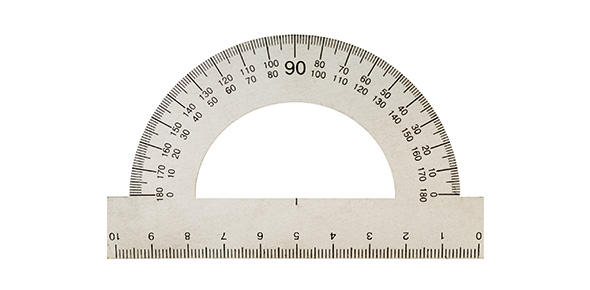# Significant Figures Quiz Questions

10 Questions | Total Attempts: 642Settings• 1.
Which rule would be used to count the amount of significant figures 500.00?
• A.

Number's Less Than One- start counting at the 1st non-zero to end

• B.

Number's Greater Than One w/ Decimal- all significant

• C.

Number's Greater Than One w/ Decimal- count only non-zeros

• D.

Number's Greater Than One w/o Decimal- exclude last place holders

• 2.
How many significant figures are in the number-     0.00320
• A.

Six

• B.

Five

• C.

Three

• D.

Two

• 3.
How many significant figures are there in the following measurement:  6000 g
• A.

One

• B.

Two

• C.

Four

• D.

None

• 4.
Calculate the sum of 6.078g and 0.3329g.
• A.

6.500 g

• B.

6.4 g

• C.

6.412 g

• D.

6.411 g

• 5.
Subtract 7.11cm from 8.2cm.
• A.

1.1 cm

• B.

1.09 cm

• C.

1.0 cm

• D.

-1.1 cm

• 6.
What is the product of 0.8102m and 3.44m?
• A.

2.787088 m^2

• B.

2.787 m^2

• C.

2.79 m^2

• D.

2.790 m^2

• 7.
Divide 94.20 g by 3.16722 mL.
• A.

29.74 g/ml

• B.

0.03362 ml/g

• C.

29.7 g/ml

• D.

0.03 ml/g

• 8.
6000 has only 1 sig fig.  Why?
• A.

It's larger than one.

• B.

It's not in scientific notation.

• C.

Its zeros are place holders.

• D.

It is rounded.

• 9.
9.0000g + 0.02g =
• A.

9.0200g

• B.

9.02 g

• C.

9 g

• D.

9.1 g

• 10.
How many significant figures in  1.00 x 10^-2
• A.

3

• B.

1

• C.

4

• D.

0

Related TopicsBack to top